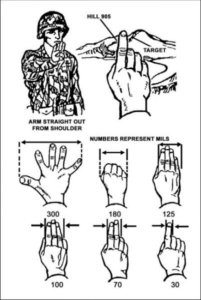Categories

# Two Methods of Estimating Distance.

A friend of mine sent me the link to this webpage.
I have not seen this particular trick before! I have not had a chance to experiment with how good an estimate this gives, so go out and have some fun with it for yourselves.
The basic method is to first select a reference object and estimate its length/width. I suggest you use yards or metres since this will give you smaller numbers to deal with and give you a more useful final answer.
Hold your right thumb at arm's length, close your right eye and align the thumb with the edge of the object.
Close your left eye and open your right. Estimate how far your thumb has “jumped” in multiples of the object length/width.
Take your answer, multiply it by the length/width in metres (or yards) and multiply that answer by ten. This will give you your estimated distance in metres or yards.
This method reminded me of one of the ways to use milliradians to estimate distance. An article on this subject can be found here. A useful thing to remember is that half a metre (a shoulder width) is one mildot at 500m.My preferred method of using milliradians is to estimate the length/width/height in metres (or yards) and divide this by the apparent measurement in mildots. (Use either a scope or your hand for the latter). This gives you a fraction, such as 2 over 1, 2 over 3, 1 over 8 etc. Multiply the fraction by 1,000 to get the distance in metres (or yards). Bone up on the decimal conversions of common fractions, such as ⅔ = 0.66 and ⅛ = 0.125.